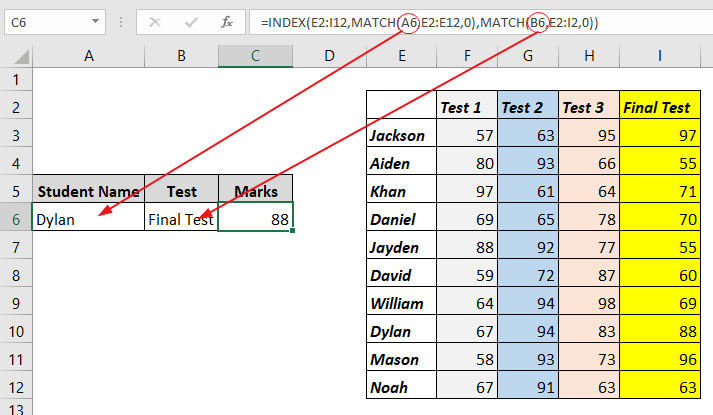What is a double lookup in Excel?

Two-way lookup or double lookup is simply a formula that looks in both vertical and horizontal directions simultaneously, in order to find a cell where a specific column and a row intersect.

If only VLOOKUP() and HLOOKUP() could be used simultaneously in a single formula, it would have been the ideal functions to perform this task. But no, these two functions can’t work together in this context.

Thus, the almighty INDEX-MATCH functions will save the day (they always do where VLOOKUP fails).

In the following data, let’s say you want to write a formula that returns a result based on two lookup values specified, one in a row and the other in a column. For instance, if you want to find the marks of David in Test 3.

See screenshot:The formula in cell B5 performs a double lookup by using the MATCH() function twice to get both the row number and the column number for the INDEX() arguments. In simple terms, the INDEX() function uses both column number and row number as arguments, which have been provided by the MATCH() function.

The match function is used twice because the formula needs to look in rows and columns (that’s in different directions).

Therefore, to write this formula, use the first MATCH() function to find the row that has David marks:

=MATCH(“David”,E2:E12,0)

Then, use the second MATCH() to find the column that has Test 3 marks:

=MATCH(“Test 3”,E2:I2,0)

Thanks to the MATCH() function, you now have the row number and the index number to be used as arguments for the INDEX() function to search the whole range of cells for the appropriate marks. So, the complete formula looks like this:

=INDEX(E2:I12,MATCH(“David”,E2:E12,0),MATCH(“Test 3”,E2:I2,0))

To make it more interesting, you can use cell referencing as lookup values in this formula so that the user can get the marks after specifying a student name and the type of test (Test 1, Test 2, Test 3, or Final Test).

See screenshot:In this illustration, you noticed that the formula is able to retrieve the marks based on student name and Test type specified in cell A6 and B6 respectively. This allows the spreadsheet user to obtain any student’s marks for any test by entering the student name plus the type of test.

The formula used here is the same as the one above. However, it uses cell referencing to specify the lookup value for the MATCH functions in the formula.

See screenshot: